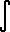Dave's Math Tables: Integral coth(x)(Math | Calculus | Integrals | Table Of | coth x)

 Discussion ofcoth x dx = ln |sinh x| + C.

## 1. Proof

Strategy: Use definition of coth; Use Substitution.  coth x = cosh x  sinh x = (ex + e-x) / 2  (ex - e-x) / 2coth x dx =ex + e-x  ex - e-x dx
set
u = ex - e-x
then we find
du = (ex + e-x) dx

substitute du= (ex + e-x) dx, u = ex - e-x
 =du  u
solve

= ln |u| + C

substitute back u = ex - e-x

= ln |ex - e-x| + C

since (ex - e-x)/2 = sinh(x)

= ln |2 sinh x| + C
= ln 2 + ln |sinh x| + C

ln 2 is merely a constant that can be combined with C

= ln |sinh x| + C
Q.E.D.